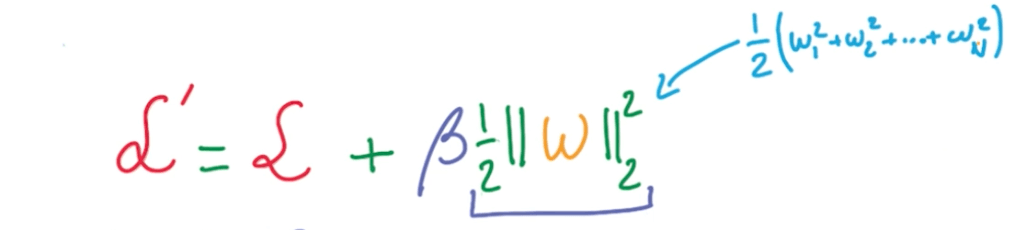# Build A Multi Layer Neural Network With L2 Regularization Using Tensorflow

For some very simple problems, a single layer neural might be able to do the job quite well . You might be able to do to process this data set with a single layer, but this is meant to show you how to build a multi layer neural network utilizing L2 regularization with Tensorflow and Python.

## Data

In this example, I am using a dataset of which gives you information about some types of mushrooms. You can find the full dataset here. I’ve reformatted this dataset so that it would be easier to use and faster to compute. You can find my version of the dataset here.

## Format the data

In the gist above, first I import the needed libraries. Those are pandas (for reading the csv file), numpy (for matrix manipulation) and Tensorflow (for the deep learning part).

Next, you’re going to need to download the dataset. Save it in the same folder as your python file. We convert the DataFrame object, which we get from pandas, to a numpy ndarray.

Now we define sizes of our datasets. The Kaglle dataset provides you with a little bit over 8,000 data points. I chose to use 5,000 of those for the training dataset and 3,000 for the validation.

After that, we need to separate the data points from the labels and delete the unnecessary variables  from memory.

## Build the graph

We will use four layers. The first layer start with 1024 nodes, and the next two have 50% of the previous layer’s node count.

We will use batches of 128 and a beta of 0.01. Beta is used in calculating the L2 loss.Define a standard Tensorflow graph. We define a functions for creating weights and biases. Biases are set to zero, and the weights are random. Next we create Tensorflow tensors for training data, labels and validation data.

I defined a functions which calculates logits for all layers of the neural network. It has a boolean parameter which is set to false by default. It determines whether will we drop some values in the matrix. When we drop values, we set its weights to 0.

L2 loss is the product of the sum of the results of the tf.nn.l2_loss function for each layer and the beta. Beta is a hyper-parameter which you need to tune to get optimal performance.

For this example I chose to use an Adam optimizer and a decaying learning rate.

At the end of this segment, we calculate predictions for the training dataset and the validations dataset.

We’ll go over the training 5001 times. I defined t a function which calculates the percentage of the accuracy.

This next section, the training, is pretty much the same as always.

We create a session, initialize all global variables. Create an offset and batch data from the said offset.

Now we just run the session and print loss and accuracy.